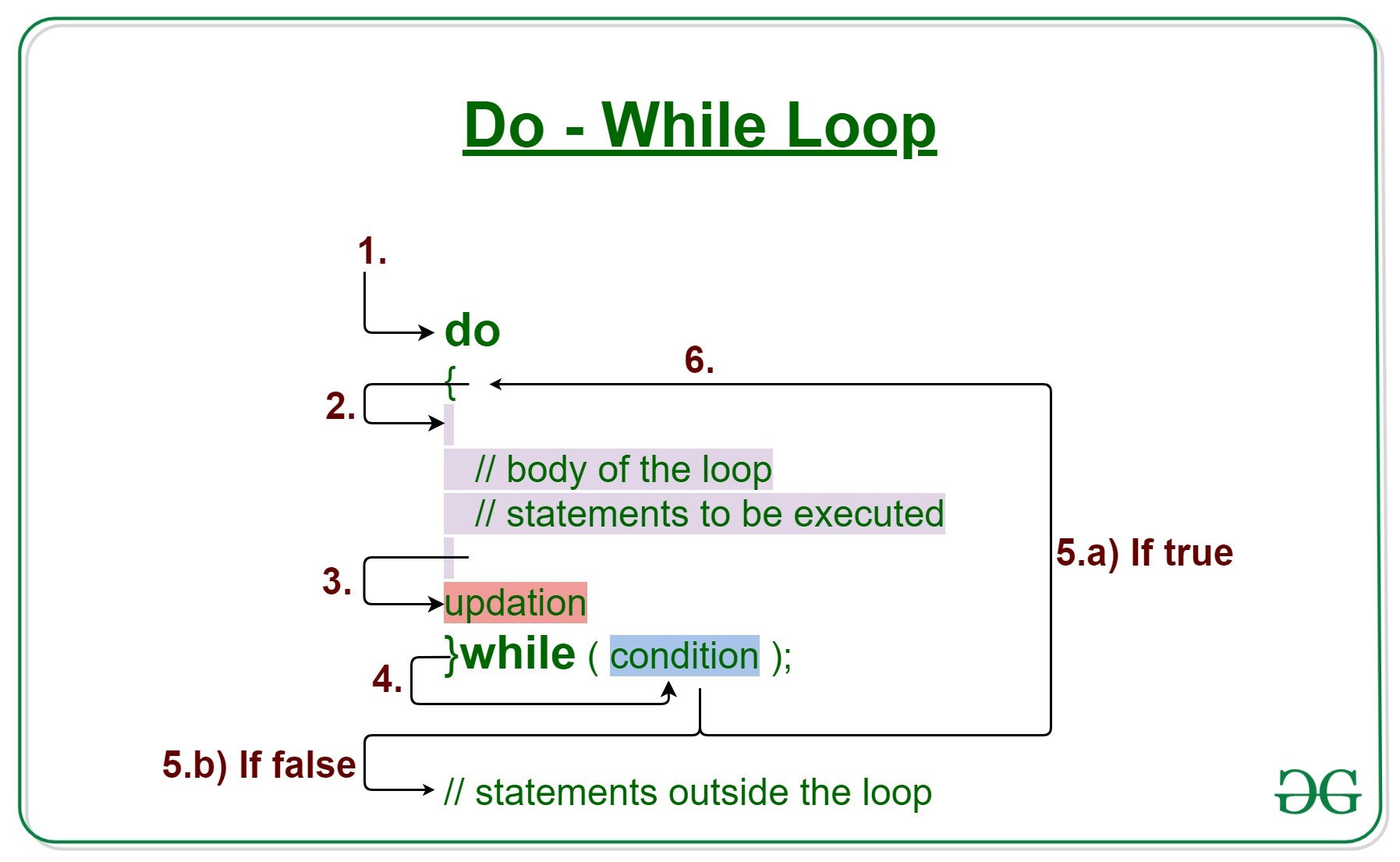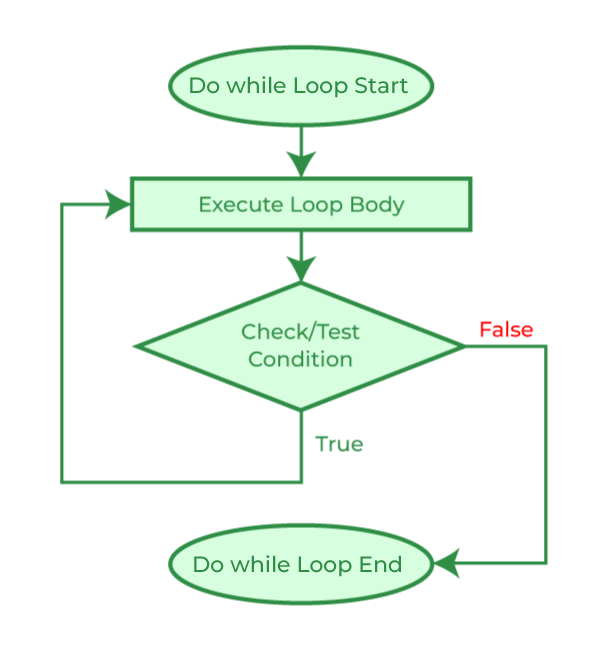Open in App
Not now

# C++ Do/While Loop

• Difficulty Level : Easy
• Last Updated : 25 Oct, 2022

Loops come into use when we need to repeatedly execute a block of statements. Like while the do-while loop execution is also terminated on the basis of a test condition. The main difference between a do-while loop and a while loop is in the do-while loop the condition is tested at the end of the loop body, i.e do-while loop is exit controlled whereas the other two loops are entry-controlled loops.

Note: In the do-while loop, the loop body will execute at least once irrespective of the test condition.Syntax:

```do
{
// loop body

update_expression;
}
while (test_expression);```

Note: Notice the semi – colon(“;”) in the end of loop.

The various parts of the do-while loop are:

1. Test Expression: In this expression, we have to test the condition. If the condition evaluates to true then we will execute the body of the loop and go to the update expression. Otherwise, we will exit from the while loop.
2. Update Expression: After executing the loop body, this expression increments/decrements the loop variable by some value.
3. Body: It is the Collection of statements i.e, variables and functions, etc. The condition is not satisfied until the condition is executed automatically after a successful iteration. do-while loop, code can be used to print simple names, execute complex algorithms, or perform functional operations.

## How does a do-While loop execute?

1. Control falls into the do-while loop.
2. The statements inside the body of the loop get executed.
3. Updation takes place.
4. The flow jumps to Condition
5. Condition is tested.
• If the Condition yields true, go to Step 6.
• If the Condition yields false, the flow goes outside the loop
6. The flow goes back to Step 2.
7. The do-while loop has been ended and flow has gone outside the loop.

## Flow Diagram of do-while loopExample 1: This program will try to print “Hello World” depending on a few conditions.

## C++

 `// C++ program to illustrate do-while loop`` ` `#include ``using` `namespace` `std;`` ` `int` `main()``{``    ``// Initialization expression``    ``int` `i = 2;`` ` `    ``do` `{``        ``// Loop body``        ``cout << ``"Hello World\n"``;`` ` `        ``// Update expression``        ``i++;`` ` `    ``}``    ``// Test expression``    ``while` `(i < 1);`` ` `    ``return` `0;``}`

Output:

`Hello World`

Dry-Run of Example 1:

```1. Program starts.
2. i is initialised to 2.
3. Execution enters the loop
a) "Hello World" gets printed 1st time.
b) Updation is done. Now i = 2.
4. Condition is checked. 2 < 2 yields false.
5. The flow goes outside the loop.```

Example 2:

## C++

 `// C++ program to illustrate do-while loop`` ` `#include ``using` `namespace` `std;`` ` `int` `main()``{``    ``// Initialization expression``    ``int` `i = 1;`` ` `    ``do` `{``        ``// Loop body``        ``cout << i << endl;`` ` `        ``// Update expression``        ``i++;`` ` `    ``}``    ``// Test expression``    ``while` `(i <= 5);`` ` `    ``return` `0;``}`

Output:

```1
2
3
4
5```

My Personal Notes arrow_drop_up